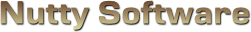Sorry, it appears you don't have support for WebGL.

In order to run this demo, you must meet the following requirements.

• You have a WebGL compatible video card with the latest drivers.
• Your video card is not blacklisted. You can check the current blacklist on Khronos.

Some browsers may require additional configuration in order to get WebGL to run. If you are having problems running this demo, visit the following sites.

#### View State

							
/// <summary>
/// </summary>

/// <summary>
/// Material source structure.
/// <summary>
struct MaterialSource
{
vec3 Ambient;
vec4 Diffuse;
vec3 Specular;
float Shininess;
vec2 TextureOffset;
vec2 TextureScale;
};

/// <summary>
/// Attributes.
/// <summary>
attribute vec3 Vertex;
attribute vec2 Uv;
attribute vec3 Normal;

/// <summary>
/// Uniform variables.
/// <summary>
uniform mat4 ProjectionMatrix;
uniform mat4 ViewMatrix;
uniform mat4 ModelMatrix;
uniform vec3 ModelScale;

uniform MaterialSource Material;

/// <summary>
/// Varying variables.
/// <summary>
varying vec4 vWorldVertex;
varying vec3 vWorldNormal;
varying vec2 vUv;
varying vec3 vViewVec;

/// <summary>
/// <summary>
void main ()
{
vWorldVertex = ModelMatrix * vec4(Vertex * ModelScale, 1.0);
vec4 viewVertex = ViewMatrix * vWorldVertex;
gl_Position = ProjectionMatrix * viewVertex;

vUv = Material.TextureOffset + (Uv * Material.TextureScale);

vWorldNormal = normalize(mat3(ModelMatrix) * Normal);

vViewVec = normalize(-viewVertex.xyz);
}


							
/// <summary>
/// This vertex shader sets up the geometry for rendering to a depth map.
/// </summary>

/// <summary>
/// Attributes.
/// <summary>
attribute vec3 Vertex;

/// <summary>
/// Uniform variables.
/// <summary>
uniform mat4 ProjectionMatrix;
uniform mat4 ViewMatrix;
uniform mat4 ModelMatrix;
uniform vec3 ModelScale;

/// <summary>
/// Varying variables.
/// <summary>
varying vec4 vPosition;

/// <summary>
/// </summary>
void main ()
{
vPosition = ViewMatrix * ModelMatrix * vec4(Vertex * ModelScale, 1.0);
gl_Position = ProjectionMatrix * vPosition;
}


							
/// <summary>
/// Vertex shader for rendering a 2D plane on the screen. The plane should be sized
/// from -1.0 to 1.0 in the x and y axis. This shader can be shared amongst multiple
/// </summary>

/// <summary>
/// Attributes.
/// <summary>
attribute vec3 Vertex;
attribute vec2 Uv;

/// <summary>
/// Varying variables.
/// <summary>
varying vec2 vUv;

/// <summary>
/// <summary>
void main ()
{
gl_Position = vec4(Vertex, 1.0);
vUv = Uv;
}


							
/// <summary>
/// </summary>

#ifdef GL_ES
precision highp float;
#endif

/// <summary>
/// Light source structure.
/// <summary>
struct LightSource
{
vec3 Position;
vec3 Attenuation;
vec3 Direction;
vec3 Colour;
float OuterCutoff;
float InnerCutoff;
float Exponent;
};

/// <summary>
/// Material source structure.
/// <summary>
struct MaterialSource
{
vec3 Ambient;
vec4 Diffuse;
vec3 Specular;
float Shininess;
vec2 TextureOffset;
vec2 TextureScale;
};

/// <summary>
/// Uniform variables.
/// <summary>
uniform int NumLight;
uniform LightSource Light;
uniform MaterialSource Material;
uniform sampler2D Sample0;

/// <summary>
/// Varying variables.
/// <summary>
varying vec4 vWorldVertex;
varying vec3 vWorldNormal;
varying vec2 vUv;
varying vec3 vViewVec;

/// <summary>
/// <summary>
void main ()
{
// vWorldNormal is interpolated when passed into the fragment shader.
// We need to renormalize the vector so that it stays at unit length.
vec3 normal = normalize(vWorldNormal);

vec3 colour = Material.Ambient;
for (int i = 0; i < 4; ++i)
{
if ( i >= NumLight )
break;

// Calculate diffuse term
vec3 lightVec = normalize(Light[i].Position - vWorldVertex.xyz);
float l = dot(normal, lightVec);
if ( l > 0.0 )
{
// Calculate spotlight effect
float spotlight = 1.0;
if ( (Light[i].Direction.x != 0.0) || (Light[i].Direction.y != 0.0) || (Light[i].Direction.z != 0.0) )
{
spotlight = max(-dot(lightVec, Light[i].Direction), 0.0);
float spotlightFade = clamp((Light[i].OuterCutoff - spotlight) / (Light[i].OuterCutoff - Light[i].InnerCutoff), 0.0, 1.0);
spotlight = pow(spotlight * spotlightFade, Light[i].Exponent);
}

// Calculate specular term
vec3 r = -normalize(reflect(lightVec, normal));
float s = pow(max(dot(r, vViewVec), 0.0), Material.Shininess);

// Calculate attenuation factor
float d = distance(vWorldVertex.xyz, Light[i].Position);
float a = 1.0 / (Light[i].Attenuation.x + (Light[i].Attenuation.y * d) + (Light[i].Attenuation.z * d * d));

colour += ((Material.Diffuse.xyz * l) + (Material.Specular * s)) * Light[i].Colour * a * spotlight;
}
}

gl_FragColor = clamp(vec4(colour, Material.Diffuse.w), 0.0, 1.0) * texture2D(Sample0, vUv);
}


							
/// <summary>
/// This fragment shader records the depth values of the geometry into a texture.
/// </summary>

#ifdef GL_ES
precision highp float;
#endif

/// <summary>
/// Uniform variables.
/// <summary>
uniform float Near;
uniform float Far;

/// <summary>
/// Varying variables.
/// <summary>
varying vec4 vPosition;

/// <summary>
/// Pack a floating point value into an RGBA (32bpp).
///
/// Note that video cards apply some sort of bias (error?) to pixels,
/// so we must correct for that by subtracting the next component's
/// value from the previous component.
/// </summary>
vec4 pack (float depth)
{
const vec4 bias = vec4(1.0 / 255.0,
1.0 / 255.0,
1.0 / 255.0,
0.0);

float r = depth;
float g = fract(r * 255.0);
float b = fract(g * 255.0);
float a = fract(b * 255.0);
vec4 colour = vec4(r, g, b, a);

return colour - (colour.yzww * bias);
}

/// <summary>
/// </summary>
void main ()
{
// Linear depth
float linearDepth = length(vPosition) / (Far - Near);

//gl_FragColor = pack(gl_FragCoord.z);
gl_FragColor = pack(linearDepth);
}


							
/// <summary>
/// This fragment shader renders the depth map to screen.
/// </summary>

#ifdef GL_ES
precision highp float;
#endif

/// <summary>
/// Uniform variables.
/// <summary>
uniform sampler2D Sample0;

/// <summary>
/// Varying variables.
/// <summary>
varying vec2 vUv;

/// <summary>
/// Unpack an RGBA pixel to floating point value.
/// </summary>
float unpack (vec4 colour)
{
const vec4 bitShifts = vec4(1.0,
1.0 / 255.0,
1.0 / (255.0 * 255.0),
1.0 / (255.0 * 255.0 * 255.0));
return dot(colour, bitShifts);
}

/// <summary>
/// <summary>
void main ()
{
float depth = unpack(texture2D(Sample0, vUv));
gl_FragColor = vec4(depth, depth, depth, 1.0);
}


							
/// <summary>
/// This fragment shader performs a DOF separable blur algorithm on the specified
/// texture.
/// </summary>

#ifdef GL_ES
precision highp float;
#endif

/// <summary>
/// Uniform variables.
/// <summary>
uniform vec2 TexelSize;		// Size of one texel (1 / width, 1 / height)
uniform sampler2D Sample0;	// Colour texture
uniform sampler2D Sample1;	// Depth texture

uniform int Orientation;	// 0 = horizontal, 1 = vertical
uniform float BlurCoefficient;	// Calculated from the blur equation, b = ( f * ms / N )
uniform float FocusDistance;	// The distance to the subject in perfect focus (= Ds)
uniform float Near;		// Near clipping plane
uniform float Far;		// Far clipping plane
uniform float PPM;		// Pixels per millimetre

/// <summary>
/// Varying variables.
/// <summary>
varying vec2 vUv;

/// <summary>
/// Unpack an RGBA pixel to floating point value.
/// </summary>
float unpack (vec4 colour)
{
const vec4 bitShifts = vec4(1.0,
1.0 / 255.0,
1.0 / (255.0 * 255.0),
1.0 / (255.0 * 255.0 * 255.0));
return dot(colour, bitShifts);
}

/// <summary>
/// Calculate the blur diameter to apply on the image.
/// b = (f * ms / N) * (xd / (Ds +- xd))
/// Where:
/// (Ds + xd) for background objects
/// (Ds - xd) for foreground objects
/// </summary>
/// <param name="d">Depth of the fragment.</param>
float GetBlurDiameter (float d)
{
// Convert from linear depth to metres
float Dd = d * (Far - Near);

float xd = abs(Dd - FocusDistance);
float xdd = (Dd < FocusDistance) ? (FocusDistance - xd) : (FocusDistance + xd);
float b = BlurCoefficient * (xd / xdd);

return b * PPM;
}

/// <summary>
/// <summary>
void main ()
{
// Maximum blur radius to limit hardware requirements.
// Cannot #define this due to a driver issue with some setups

// Pass the linear depth values recorded in the depth map to the blur
// equation to find out how much each pixel should be blurred with the
// given camera settings.
float depth = unpack(texture2D(Sample1, vUv));
float blurAmount = GetBlurDiameter(depth);

// Apply the blur
float count = 0.0;
vec4 colour = vec4(0.0);
vec2 texelOffset;
if ( Orientation == 0 )
texelOffset = vec2(TexelSize.x, 0.0);
else
texelOffset = vec2(0.0, TexelSize.y);

if ( blurAmount >= 1.0 )
{
float halfBlur = blurAmount * 0.5;
for (float i = 0.0; i < MAX_BLUR_RADIUS; ++i)
{
if ( i >= blurAmount )
break;

float offset = i - halfBlur;
vec2 vOffset = vUv + (texelOffset * offset);

colour += texture2D(Sample0, vOffset);
++count;
}
}

// Apply colour
if ( count > 0.0 )
gl_FragColor = colour / count;
else
gl_FragColor = texture2D(Sample0, vUv);
}


							
/// <summary>
/// This fragment shader produces the final image with the DOF effect. The blurred image is
/// blended with the higher resolution texture based on how much each pixel is blurred.
/// </summary>

#ifdef GL_ES
precision highp float;
#endif

/// <summary>
/// Uniform variables.
/// <summary>
uniform sampler2D Sample0; // Colour texture
uniform sampler2D Sample1; // Depth texture
uniform sampler2D Sample2; // Blurred texture

uniform float BlurCoefficient;	// Calculated from the blur equation, b = ( f * ms / N )
uniform float FocusDistance;	// The distance to the subject in perfect focus (= Ds)
uniform float Near;		// Near clipping plane
uniform float Far;		// Far clipping plane
uniform float PPM;		// Pixels per millimetre

/// <summary>
/// Varying variables.
/// <summary>
varying vec2 vUv;

/// <summary>
/// Unpack an RGBA pixel to floating point value.
/// </summary>
float unpack (vec4 colour)
{
const vec4 bitShifts = vec4(1.0,
1.0 / 255.0,
1.0 / (255.0 * 255.0),
1.0 / (255.0 * 255.0 * 255.0));
return dot(colour, bitShifts);
}

/// <summary>
/// Calculate the blur diameter to apply on the image.
/// b = (f * ms / N) * (xd / (Ds +- xd))
/// Where:
/// (Ds + xd) for background objects
/// (Ds - xd) for foreground objects
/// </summary>
/// <param name="d">Depth of the fragment.</param>
float GetBlurDiameter (float d)
{
// Convert from linear depth to metres
float Dd = d * (Far - Near);

float xd = abs(Dd - FocusDistance);
float xdd = (Dd < FocusDistance) ? (FocusDistance - xd) : (FocusDistance + xd);
float b = BlurCoefficient * (xd / xdd);

return b * PPM;
}

/// <summary>
/// <summary>
void main ()
{
// Maximum blur radius to limit hardware requirements.
// Cannot #define this due to a driver issue with some setups

// Get the colour, depth, and blur pixels
vec4 colour = texture2D(Sample0, vUv);
float depth = unpack(texture2D(Sample1, vUv));
vec4 blur = texture2D(Sample2, vUv);

// Linearly interpolate between the colour and blur pixels based on DOF
float blurAmount = GetBlurDiameter(depth);
float lerp = min(blurAmount / MAX_BLUR_RADIUS, 1.0);

// Blend
gl_FragColor = (colour * (1.0 - lerp)) + (blur * lerp);
}



# Depth of Field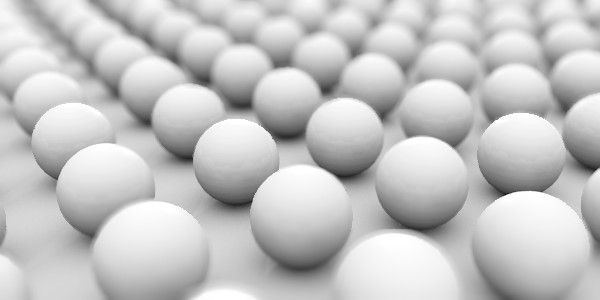## Introduction

Depth of field (abbr. DOF) is a region where subjects appear to be in focus. When a subject moves away from the point of focus, it begins to defocus or blur due to the physical nature of how light interacts with lenses (including your eyes) and projects an object onto a screen. In computer graphics, you don't have to deal with the physical limitations of reality. You do not view the world from a lens, but often deal with the mathematics that render graphics from a single point that provides an infinite DOF. In some cases this is a desired effect, in other cases it's nice to add the realistic deficiencies that comes with viewing through a real camera. The goal of this article is to explain DOF and how you can implement it into your games to produce realistic looking images.

## Why DOF?

DOF is a popular technique in photography because we tend to naturally observe things in focus first. Video games use DOF for the same reasons. Using DOF can help you grab gamers attention by focusing their eyes to a particular point on the screen. This can be useful when you want the player to follow character narration during a cinematic sequence or if you want to raise the player's awareness of an incoming threat.

Some care should be taken to avoid overusing DOF. While it's a powerful tool to grab someone's attention, it can quickly become a nuisance if you incorporate it into live gameplay. You need to find the right balance between realism and enjoyability.

## What is DOF?

In computer graphics, there's no such thing as a camera lens. All game objects render with pin-point accurate focus. That is at least how the mathematics of a standard renderer tends to work. This is illustrated below.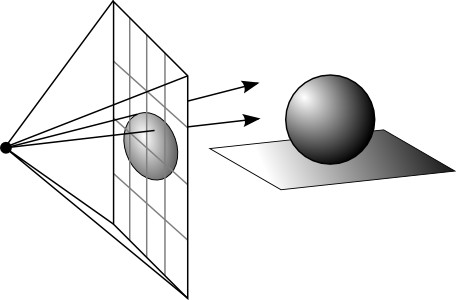Figure 1. Computer rendered graphics.

The camera lens in this case is a tiny point, also called a pinhole camera in the photography world. Pinhole cameras essentially produce a direct beam of light onto the viewscreen, producing an infinite DOF. Each pixel on the viewscreen maps to a specific object colour in the game world. Whether you render graphics using a raytracer or a rasterizer, the fact remains that one pixel is one colour. The focus is always on the polygon being rendered, just like in a pinhole camera. In real life however, a camera lens is larger than a point and must be able to focus the light on the viewscreen. The larger the lens and aperture (aperture is the size of the hole to allow light into the camera), the greater the focal length (ie: camera zoom), the more shallow the DOF and thus greater potential for blurry images. This is illustrated below.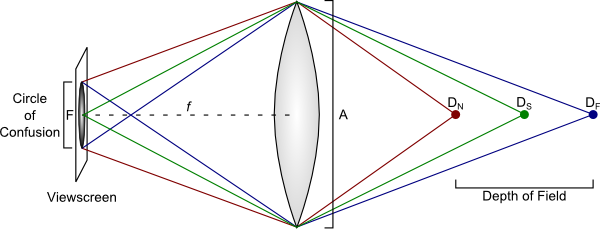Figure 2. Light projection from a camera and its lens.

Where

$D_N$ is the nearest distance a subject will remain in focus.

$D_S$ is the distance to the subject in perfect focus.

$D_F$ is the farthest distance a subject will remain in focus.

$F$ is the focal point. This is the point where light converges onto a single point on the viewscreen, producing the best possible focus.

$f$ is the focal length. This is the distance between the viewscreen and the lens.

$A$ is the lens diameter. Normally you represent the diameter as a focal ratio, also called an f-number or f-stop. This allows us to work with one less piece of information. For example, a 100mm focal length with an f-stop of f/2.8 has an aperture diameter of (100 / 2.8) = 35.7mm.

In figure 2, a lens focuses the light entering the camera from the right onto the viewscreen on the left. An object is considered in focus when the beams of light intersect exactly on the viewscreen. This is illustrated with the green line and subject at distance $D_S$. Objects between $D_N$ and $D_F$ will also appear to be in focus, even though the light doesn't converge exactly on the viewscreen. This area is called the "Circle of Confusion". Why is this so? This is because our vision is not perfect. We cannot discern small amounts of blurring, but we will eventually start to see it once it grows in size. This will start to happen when light diverges away from the circle of confusion. Or in other words, when an object is outside the DOF.

Another way to look at the circle of confusion is in terms of pixels. A pixel has a 1x1 area. Imagine the focus of a point has a blur radius of 0.1. The net effect on the pixel is irrelevant as the blur radius is significantly smaller than the pixel itself. It is therefore acceptable to just colour the pixel as normal.

## Implementing DOF

There are two ways you can go about implementing DOF. One way involves little math. You simply provide $D_N$ and $D_F$ to a shader and if a fragment is outside of this range, you blur it accordingly.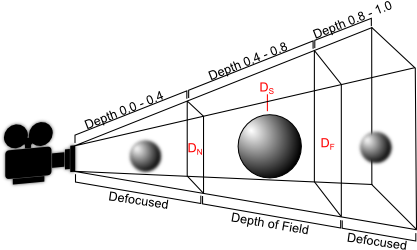Figure 3. DOF implementation using depth ranges.

While easy to implement, it's difficult to manage. Knowing the exact ranges you want to blur and doing it within a realistic manner while zooming in a camera is quite cumbersome. The other approach, and the one used by the WebGL demo, is to calculate the blur radius using actual camera properties such as the focal length, the f-stop, and the distance to the subject in focus. This requires implementing some lens equations, but it makes working with DOF easier by using properties most would be familiar with when behind a real camera.

The first equation we'll look at is the blur diameter equation. This equation solves how much a pixel is blurred based on its distance from the camera.

$b = \frac{f m_s}{N} \frac{x_d}{D_S \pm x_d}$

Where

$f$ is the focal length.

$m_s$ is the subject magnification $m_s = \frac{f}{D_S - f}$.

$N$ is the f-stop.

$x_d$ is the distance from the subject ($D_S$) to the distance of the fragment $x_d = |D - D_S|$.

$D_S$ is the distance to the subject in perfect focus.

$b$ is the calculated blur disc diameter. Objects in the foreground use subtraction $D_S - x_d$ and objects in the background use addition $D_S + x_d$.

For example, let's solve the blur disc diameter for a 35mm camera with a focal length of 50mm, an f-stop of 2.8, a subject focus distance of 10 metres, and a viewscreen with a resolution of 1024 x 768 pixels. From this, we calculate $b$.

$f = 50mm$ $D_S = 10000mm$ $m_s = \frac{50}{10000 - 50}\ = 0.005$ $N = {2.8}$ $b = \frac{50 * 0.005}{2.8} \frac{x_d}{10000 \pm x_d} = 0.089 \frac{x_d}{10000 \pm x_d}$

Now, let's assume we have an object 2m (2000mm) in front of the camera.

$x_d = |2000 - 10000| = 8000$ $b = 0.089 \frac{8000}{10000 - 8000} = 0.356mm$

To convert the blur disc diameter into pixels, we need to find the pixels per millimetre (abbr. PPM) of the viewscreen. A 35mm frame at a resolution of 1024 x 768 pixels has a PPM of: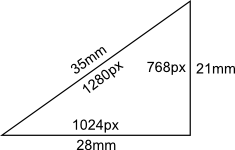1px / 1mm = 1280px / 35mm = 36.57 px/mm. A blur with a diameter of 0.356mm will blur an area of 0.356mm * 36.57px/mm = 13 pixels. If you halve the f-stop, you also halve the blur area. An f-stop of 5.6 will blur an area of 6.5 pixels. If you wanted an object 2 metres from the camera to be in focus, you would need to produce a blur diameter less than 1 / 36.57px/mm, which also happens to be the size of the circle of confusion. This works out to an f-stop of about 37. This is solved by substituting the values back into the blur equation and solving for N.

## Blurring

The separable blurring technique (also known as the box-blur) gets its name from the way it performs blurring. In the traditional sense, blurring is performed using a convolution filter. That is, an N x M matrix that samples neighbouring pixels and finds an average. A faster way to perform this activity is to separate the blurring into two passes. The first pass will blur all pixels horizontally. The second pass will blur all pixels vertically. The result is the same as performing blur with a convolution filter at a significantly faster speed.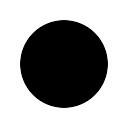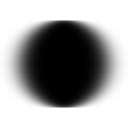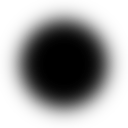Left: Unfiltered image.

Middle: Pass 1, horizontal blurring applied.

Right: Pass 2, vertical blurring applied.

What's unique here is that the lower resolution you use, the more effective the blurring. A 256x256 texture for instance can produce a very soft blur with a small blur radius, whereas a 1024x1024 texture requires a large kernel to blur it sufficiently enough. You have to find the right balance between resolution and blurring. To much of either can hinder performance.

## Blending the final results

Once you generate the low resolution blur, the next step is to blend it with the high resolution scene render. The pixels that are defocused will take from the lower resolution blurred texture, and the pixels that are in focus will take from the higher resolution texture. Pixels that are somewhat blurred will linearly interpolate between the high resolution and low resolution blur texture so that the image quality isn't degraded to rapidly.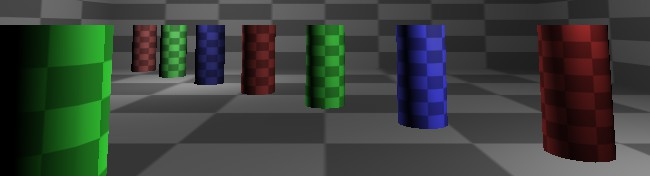Figure 4a. High resolution scene render.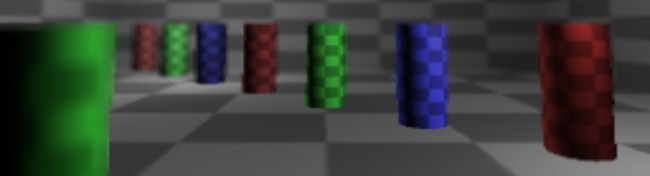Figure 4b. Low resolution DOF blur.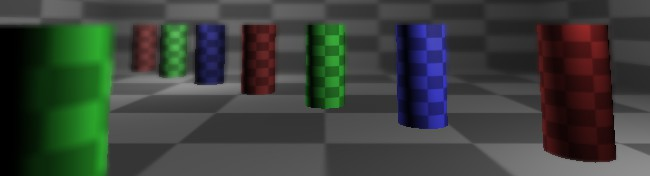Figure 4c. Blended result.

To interpolate between the textures, use the blur disc diameter equation to determine how much a pixel should be blurred. The closer that value is to the maximum blur radius, the more you sample from the low resolution blur texture. The following code snippet is taken from the dof_image fragment shader.

	
// Get the colour, depth, and blur pixels
vec4 colour = texture2D(Sample0, vUv);
float depth = unpack(texture2D(Sample1, vUv));
vec4 blur = texture2D(Sample2, vUv);

// Linearly interpolate between the colour and blur pixels based on DOF
float blurAmount = GetBlurDiameter(depth);
float lerp = min(blurAmount / MAX_BLUR_RADIUS, 1.0);

// Blend
gl_FragColor = (colour * (1.0 - lerp)) + (blur * lerp);



Note that in doing this you will cut off some blur from the DOF, but it produces a nice smooth transition between the high resolution and lower resolution images. You can hasten the interpolation by adding a multiplier into the equation, so that blurring occurs more quickly. Just make sure there's enough fall-off between the high resolution and low resolution texture to avoid the scissoring effect (similar to screen tearing when you disable vsync). It's not something that can be easily seen from a still image, but when you animate the DOF you will notice it quite clearly.

## References

1. Wikipedia Editors (2012-04-29). “Depth of field”. Wikipedia. Retrieved 2012-05-11.

The source code for this project is made freely available for download. The ZIP package below contains both the HTML and JavaScript files to replicate this WebGL demo.

The source code utilizes the Nutty Open WebGL Framework, which is an open sourced, simplified version of the closed source Nutty WebGL Framework. It is released under a modified MIT license, so you are free to use if for personal and commercial purposes.